# Comparison of the Experimental and Predicted Data for Thermal Conductivity of Fe3O4/water Nanofluid Using Artificial Neural Networks

Document Type : Original Research Article

Authors

1 Young Researchers and Elite Club, Shahrood Branch, Islamic Azad University, Shahrood, Iran

2 Department of Chemistry, Sciences Faculty, Arak Branch, Islamic Azad University, Arak, Iran

3 Department of Chemical Engineering, Islamic Azad University, Saveh Branch, Saveh, Iran

4 Department of Mechanical Engineering, Shahrood branch, Islamic Azad university, Shahrood, Iran

Abstract

Objective(s): This study aims to evaluate and predict the thermal conductivity of iron oxide nanofluid at different temperatures and volume fractions by artificial neural network (ANN) and correlation using experimental data.

Methods: Two-layer perceptron feedforward artificial neural network and backpropagation Levenberg-Marquardt (BP-LM) training algorithm are used to predict the thermal conductivity of the nanofluid. Fe3O4 nanoparticles are prepared by chemical co-precipitation method and thermal conductivity coefficient is measured using 2500TPS apparatus.
Results: Fe3O4 nanofluids with particle size of 20-25 nm are used to test the effectiveness of ANN. Thermal conductivity of Fe3O4 /water nanofluid at different temperatures of 25, 30 and 35℃ and volume concentrations, ranging from 0.05% to 5% is employed as training data for ANN. The obtained results show that the thermal conductivity of Fe3O4 nanofluid increases linearly with volume fraction and temperature.

Conclusions: the artificial neural network model has a reasonable agreement in predicting experimental data. So it can be concluded the ANN model is an effective method for prediction of the thermal conductivity of nanofluids and has better prediction accuracy and simplicity compared with the other existing theoretical methods.

Keywords

#### Full Text

INTRODUCTION

Although there are many different methods for heat transfer enhancement, low thermal conductivity of the typical fluids reduces the efficiency of the heat exchangers. Improving thermal properties of these fluids in energy transfer can increase the heat transfer. Mixing small solid particles into the fluids such as water, ethylene glycol, and oil is an innovative method for improving the thermal conductivity of these fluids. Different metallic and nonmetallic powders and polymeric particles can be added to the fluids to form a suspension. According to the obtained results, the thermal conductivity of the fluids containing suspended particles is higher than that of the typical fluids. Liu et al. have investigated the effects of the load resulting from particles concentration, size and flow rate on the pressure loss of this slurry and its heat transfer behavior. In recent researches, micro- and millimeter scales have been used. These coarse particles can cause severe problems such as abrasion and blockade of the channel. Therefore, addition of the large dispersed particles does not increase the heat transfer considerably and it is not economical . Nanoparticles can improve properties and enhance the heat transfer of the fluids. Particles with the diameter below 100nm show different properties from the common solid particles. Compared to the micron particles, nano-powders have larger surface area and higher potential for enhancing heat transfer. Many researchers have tried to produce a high effective heat transfer potential fluid by dispersing nanoparticles in it. Results obtained from the early experiments like the study of Eastman et al. showed a 60% increase in the thermal conductivity of 5 % (v) nanofluids containing water and CuO nanoparticles . Dispersion of nanoparticles into the cooling or heating fluid can cause a significant increase in the heat transfer. This increase can be attributed to the following possible reasons:

Dispersed nanoparticles increase the surface area and thermal capacity of the fluid.

Dispersed nanoparticles improve the effective or apparent thermal conductivity of the fluid.

Contacts between particles, fluid, and surface of the passing flow increase the interactions.

Turbulence of the fluid and mixture increase.

Dispersion of nanoparticles levels the diagonal temperature gradient of the fluid.

When it comes to the stability of the suspension, it has been shown that sedimentation of particles can be prevented by utilizing proper dispersants. Studies regarding the thermal conductivity of nanofluids have showed that high enhancements of thermal conductivity can be achieved by using nanofluids. It is possible to obtain thermal conductivity enhancements larger than 20% at a particle volume fraction smaller than 5% . Such enhancement values exceed the predictions of theoretical models developed for suspensions with larger particles. This is considered as an indication of the presence of additional thermal transport enhancement mechanisms of nanofluids. There are many experimental and theoretical studies in the literature regarding the thermal conductivity of nanofluids. In thermal conductivity measurements of nanofluids, the transient hot-wire technique is the most commonly used method. A modified transient hot-wire method is required in the measurements, since nanofluids conduct electricity. The modification is made by insulating the wire. Some other methods such as steady-state parallel-plate technique, temperature oscillation technique, microhot strip method, and optical beam deflection technique have also been utilized by some researchers [4-9]. Maddah et al.  have investigated the thermal and electrical conductivity and viscosity of Al2O3 and Ag nanoparticles dispersed in the water at various volume concentrations, ranging from 0.25% to 5% at a temperature of 15°C. They have found a higher increase in the electrical conductivity and viscosity of nanofluids than their thermal conductivity compared to the base fluid. Artificial neural networks are used for predicting the thermal conductivity of nanofluids. Recently, many researchers have conducted various investigations in this regard and their results are acceptable. Hemmat Esfe et al.  have presented an experimental investigation on the thermal conductivity of ZnO–EG nanofluid. They have utilized ZnO nanoparticles with the size of 18nm dispersed in the different volume fractions of ethylene glycol and different temperatures ranging from 24 to 50. In their study, they have used a multi-layer perceptron feedforward neural network for modeling the thermal conductivity of ZnO–EG nanofluid. Out of 40 data obtained from experiments, 28 data were selected for network training, while the remaining 12 data were used for network test and validation. Their results have shown that the outputs of the artificial neural network have a good agreement with the experimental results.

Tajik Jamal-Abadi and Zamzamian  have studied, optimized and reported the effects of various parameters such as the ratio of the thermal conductivity of nanoparticles to that of a base fluid, volume fraction, nanoparticle size, and temperature on the effective thermal conductivity of nanofluids using nonlinear optimization methods and artificial neural network. The results for nonlinear optimization methods showed that thermal conductivity of nanofluid enhanced by 32 percent. For the modeling of the thermal conductivity of nanofluid, the feed-forward back-propagation ANN is employed. Results showed the maximum enhancement of 42 percent for thermal conductivity and also, this method is more acceptable since excellent agreement between the predictions and the experimental data is obtained with a MAE (mean absolute error) of 0.30%. In the present study, the obtained results from measurements of thermal conductivity of Fe3O4 in different temperatures and concentrations were evaluated and compared with data obtained from artificial neural networks. Temperature and concentration were used as inputs and thermal conductivity is used as target for ANNs. In addition, the predicted data by ANNs were compared to experimental data. Finally, error difference between predicted and experimental data were also investigated.

MATERIALS AND METHODS

Fe3O4 Nanoparticles

Fe3O4 nanoparticles used in this study were 20-25nm in size. First, Fe3O4 nanoparticles were prepared by chemical co-precipitation method.  Then, Fe3O4nanoparticles were weighted for each volume concentration and then, added into the water (base fluid). After that, the mixture was stirred for 60 min using a mechanical stirrer. Next, the suspension was put inside an ultrasonic vibrator for 4h. By utilizing the stirrer and ultrasonic vibrator, the sample was homogeneously stirred in the way that no sedimentation and agglomeration was observed with the naked eyes for a long period. Fig. 1 shows SEM image of the nanoparticles used in this study. Fe3O4 nanoparticles have higher surface area. Moreover, they have a high capability in increasing the heat transfer and producing stable suspension.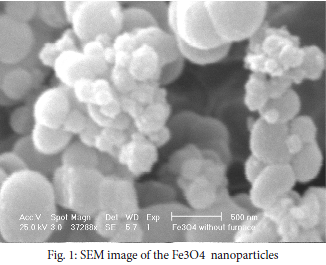Measuring  thethermal conductivity coefficient of the Fe3Onanofluid

Thermal conductivity coefficient was measured using 2500 TPS apparatus (Hot Disk Co., Gothenburg, Sweden; see Fig. 2) The apparatus performed on the basis of TPS method in which a planar sensor and mathematical model are used to describe thermal conductivity coefficient. These two parameters together with electronic equipment made the apparatus able to measure the heat transfer of material. The sensor consists of a thin twisted tape between two kepton plastic layers subjected to the considered material. Kepton layers acted as an electric insulator and increased the mechanical strength of the sensor. Additionally, during the measurement process, a fluid flow such as air or water entered into the twisted tape and increased the temperature. Heat generated between the sample in the apparatus and two sides of the sensor varied depending on the thermal properties of the studied material. The properties of the tested material were determined according to the recorded temperatures against the reaction time. The ultrasonically dispersed and homogenized Fe3O4 nanofluid was prepared to inject into the metal box and put into the water bath to reach the considered temperature. Once the nanofluid became stable, the measurement was done by Hot Disk requiring the temperature dependent parameters. The thermal conductivity of the Fe3O4 nanofluid (0.05%-5 % (v/v)) was measured.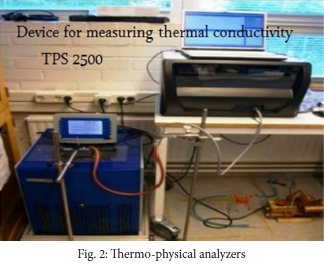Artificial neural networks (ANNs) are simple statistical models inspired by biological neural network, which perform functions in parallel. After regulating or training the neural network, it generates a desired response by receiving one or more special inputs. In supervised neural networks, there is a match between input and target values and finally, the output values and target function match, too. Neural networks are used to solve different complex functions. ANNs are applied to predict the output values of different processes. So, the proper selection of input data can generate the desired output value. Initially, the desired data and target values are given to the software for prediction of the output values of neural network. Multi-layer perceptron (MLP) feedforward neural network usually has one or more hidden layers, in which prediction depends on the selection of suitable numbers of neurons for each layer. Neurons in each layer are connected to other neurons by mass coefficients. Outputs of neurons are calculated by an activation functions used to determine the sum of initial weights and orientation of each neuron. In general, the following steps are followed in an artificial neural network:

Generation of the training data for the network.

Evaluation of the different structures for selecting the optimal structure.

Testing the neural network using the unused data in network training.

Oriented neurons are connected to the neurons of next layers to form a fixed direction. The most exact and proper predictions are obtained by tangent function for hidden layer and by pure linear function for output layer. Fig. 3 represents a neural network with two hidden layers consisting of 10 neurons and one output layer. In training algorithms, training data points are inputs and network repeatedly updates the weights and orientations until there is a good agreement between predicted and desired values. In this work, different temperatures and volume fractions of nanoparticles are used as input data and the thermal conductivity coefficients of Fe3O4 nanofluid obtained from experiments are the target or output data. Different topologies and different numbers of neurons were used to investigate the performance of the multi-layer perceptron neural network. In the first step, termination of training networks was done when mean squared error (MSE) was calculated. From all data points, 70% data were used for network training, while the remaining 30% data were utilized for network test and validation. Since the number of neurons in hidden layer is important, a sensitivity analysis was conducted on this parameter. MSE and R were used to assess the obtained results.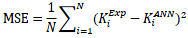(1)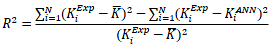(2)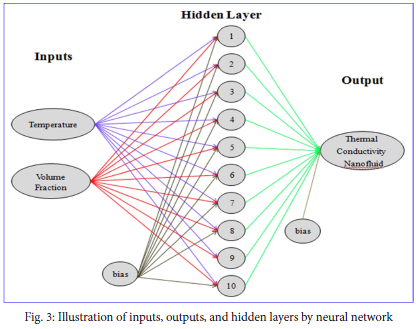According to the obtained results, the neural networks have a good ability for predicting thermal conductivity coefficient ratio. As mentioned earlier, two-layer perceptron feedforward neural network and backpropagation Levenberg-Marquardt (BP-LM) training algorithm were used to predict the thermal conductivity of the nanofluid. Volume fraction and temperature were utilized as inputs and thermal conductive coefficient was used as output (target) parameter. Fig. 3 represents the scheme of structure of the neural network. The number of neurons in hidden layers requires the sensitivity analysis. Therefore, this parameter was evaluated using trial and error method. In this study, hidden layer had 10, 15, and 20 neurons and their results were shown, individually. Using a large number of neurons can make the run time longer and increase the probability of error path in the model. 10 neurons have abnormal error distribution.

RESULT AND DISCUSSION

Fig. 4(a, b and c) shows the thermal conductivity of Fe3O4/water nanofluid at different volume fractions and different temperatures (25, 30 and 35 °C). The obtained results showed that the thermal conductivity of nanofluids increases linearly with volume fraction and temperature. For example, thermal conductivity ratio of Fe3O4 nanofluid in temperatures 25, 30 and 35 °C is 0.643, 0.65693 and 0.6834, respectively. Increased thermal conductivity can be attributed to the existence of dispersed particles. As seen in Fig. 4 (a-c), thermal conductivity coefficient increases with temperature. This would be a result of an increase in Brownian motion and the contacts of particles.  The thermal conductivity of nanofluid strongly depends on the volume fraction. Although, there are semi-empirical correlations for calculating the apparent conductivity of two-phase mixtures, one of the unsolvable problems is the appearance of nonrealistic theories for prediction of nanofluids thermal conductivity. Random migration of nanoparticles is the main reason of increasing the thermal conductivity of nanofluids, because the volume fraction of nanoparticles is so small therefore, such effects are not observed in average volume or average weight. Fine particles have large surface area. Heat transfer between fluid and particle occurs in their interface. Thus, nanoparticles having large surface area increase the thermal conductivity.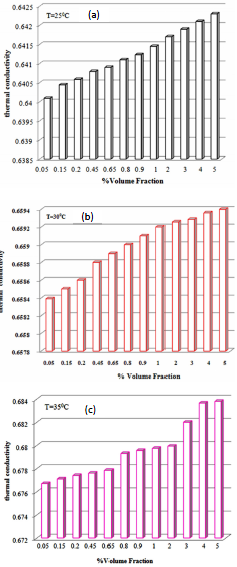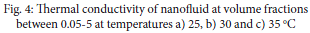Fig. 5 shows the comparison between experimental and predicted results for thermal conductivity. The number of neurons in hidden layer is 10 (Fig. 5a), 15 in (Fig. 5b) and 20 in (Fig. 5c). 10 neurons gave a higher MES. The maximum error value decreases with an increase in the number of neurons, while the correlation coefficient increases. Thus, it can be concluded that there is a good agreement between the predicted and experimental results. This regression value confirms good performance of the network. It is clear in the Fig. 5 that the experimental values agree with the model outputs.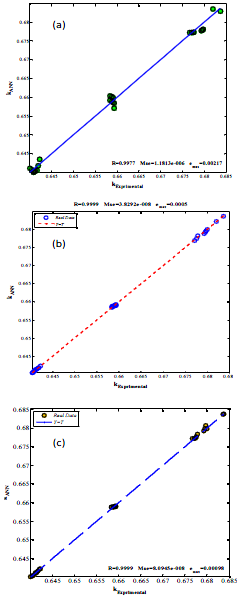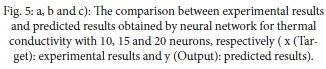As shown in Fig. 6, the number of the tested samples by the neural network is 36. According to this Figure, there is a good agreement between the thermal conductivity ratios obtained by the neural network and the experimental results. It is clear in Fig. 7 that each sample has one minimum and one maximum error value. According to Fig 7(a), the maximum and minimum error values are not the same. In Figs. 7(b) and (c), the maximum and minimum error values are for the samples 12, 33 and 36, respectively. Therefore, it can be mentioned that the prediction accuracy increases with the number of neurons. The most accurate results were obtained by increasing the number of neurons in hidden layer or the number of training vectors. The number of inputs or their values can be increased. In this work, the maximum error and correlation coefficient values for selected 20 neurons were equal to 0.00098 and 0.99999, respectively.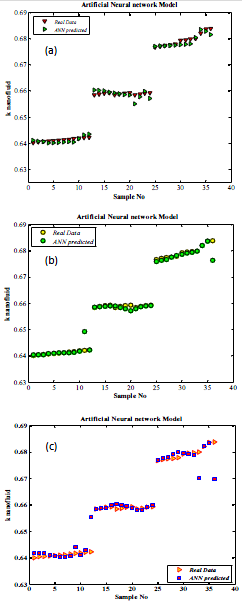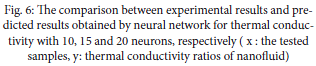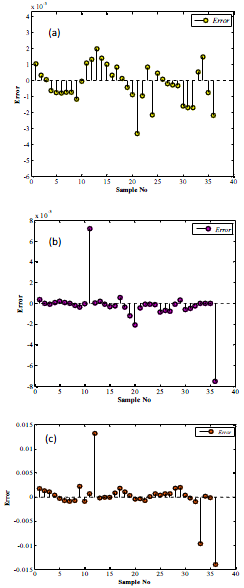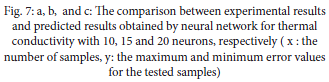Fig. 8 shows the thermal conductivity ratio of Fe3O4 nanofluid at different volume fractions. The thermal conductivity of the nanofluid increases with the temperature and volume fraction. As represented in Fig. 8, the thermal conductivity of nanofluid was investigated at temperatures 25 and 30 °C and volume fractions between 0.05-5. Then, the predicted results obtained by neural network for thermal conductivity were compared with the experimental results. According to this figure, there is a good agreement between experimental and predicted results.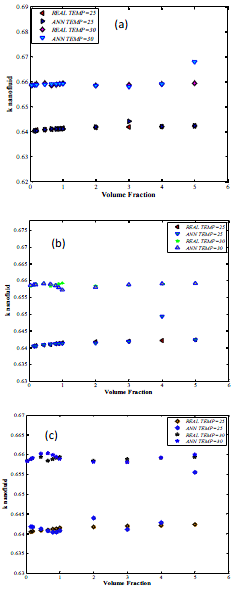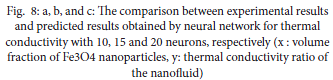Tajik Jamal-Abadi and Zamzamian  have modeled the thermal conductivity coefficient of Al2O3 nanofluid using ANN. They have studied nanoparticle size but ignored the maximum error values. In the present study, the maximum error values and utilization of Fe3O4 nanofluid were considered. The temperatures used in our study were different. In another work, Ariana etal.  have reported the modeling of the thermal conductivity coefficient of Al2O3 nanufluid using ANN. The number of neurons in their study was 40 but in the present study numbers of neurons used for training were 15, 10 and 20, respectively, to select the best number of neurons with minimum error. Hemmat Esfe et al.  have used tansig function as the transfer function in the training process of network but in the present study the effectiveness of network was evaluated using functions tansig, logsig and purelin. In addition, nanofluid used in their study was MgO–water/EG with the volume ratio of 60:40.

CONCLUSION

In the present study, thermal conductivity of Fe3O4 nanofluid was investigated experimentally. Then, the artificial neural network was used to predict the thermal conductivity. Volume fraction and temperature were considered as network inputs and thermal conductivity ratio as output. The obtained results showed that there is a good agreement between the predicted and experimental results and the sum of MES and R values  are  desirable.

ACKNOWLEDGMENTS

The author would like to thank Nano Technology Institution, Islamic Azad University of Saveh and also to thank F. Ashori for his helpful comments, which has helped the author in improving the quality of this manuscript.

Conflict of interest

The authors declare that there are no conflicts of interest regarding the publication of this manuscript.

#### References

1.Liu K, Choi U, Kasza KE. Measurements of pressure drop and heat transfer in turbulent pipe flows of particulate slurries. Argonne National Lab., IL (USA), 1988.
2.Eastman JA, Choi SUS, Li S, Thompson LJ, Lee S. Enhanced thermal conductivity through the development of nanofluids. MRS Symp Proc. 1996;457.
3.Eastman JA, Choi SUS, Li S, Yu W, Thompson L. Anomalously increased effective thermal conductivities of ethylene glycol-based nanofluids containing copper nanoparticles. Appl Phys Lett. 2001;78(6):718-20.
4.Yu W, France DM, Routbort JL, Choi SUS. Review and comparison of nanofluid thermal conductivity and heat transfer enhancements. Heat Transfer Eng. 2008;29(5):432-60.
5.Chon CH, Kihm KD, Lee SP, Choi SUS. Empirical correlation finding the role of temperature and particle size for nanofluid (Al2O3) thermal conductivity enhancement. Appl Phys Lett. 2005;87(15):3107.
6.Das SK, Putra N, Thiesen P, Roetzel W. Temperature dependence of thermal conductivity enhancement for nanofluids. J Heat Transfer. 2003;125(4):567-74.
7.Li CH, Peterson G. Experimental investigation of temperature and volume fraction variations on the effective thermal conductivity of nanoparticle suspensions (nanofluids). J Appl Phys. 2006;99(8):084314.
8.Hwang KS, Jang SP, Choi SUS. Flow and convective heat transfer characteristics of water-based Al2O3 nanofluids in fully developed laminar flow regime. Int  J Heat Mass Transfer. 2009;52(1):193-9.
9.Heris SZ, Esfahany MN, Etemad SG. Experimental investigation of convective heat transfer of Al2O3/water nanofluid in circular tube. Int J Heat Fluid Fl. 2007;28(2):203-10.
10.Esfe MH, Saedodin S, Naderi A, Alirezaie A, Karimipour A, Wongwises S, et al. Modeling of thermal conductivity of ZnO-EG using experimental data and ANN methods. Int Commun Heat Mass. 2015;63:35-40.
11.Tajik Jamal-Abadi M, Zamzamian A. Optimization of thermal conductivity of Al2O3 nanofluid by using ANN and GRG methods. Int J Nanosci  Nanotechnol. 2013;9(4):177-84.
12.Ariana M, Vaferi B, Karimi G. Prediction of thermal conductivity of alumina water-based nanofluids by artificial neural networks. Powder Technol. 2015;278:1-10.
13.Esfe MH, Rostamian H, Afrand M, Karimipour A, Hassani M. Modeling and estimation of thermal conductivity of MgO–water/EG (60: 40) by artificial neural network and correlation. Int Commun Heat Mass. 2015;68:98-103.